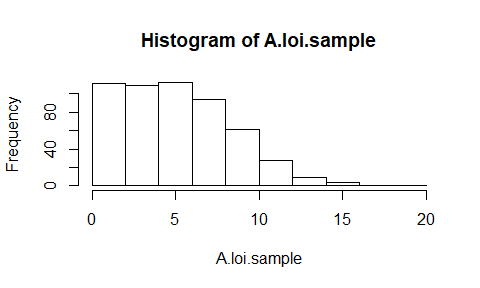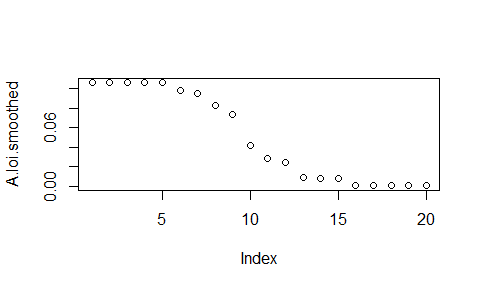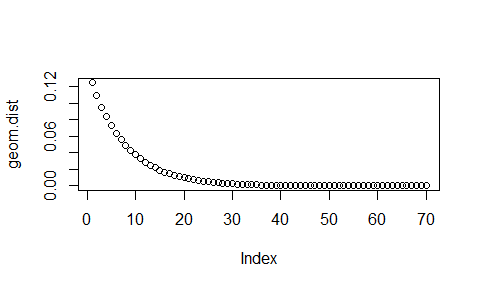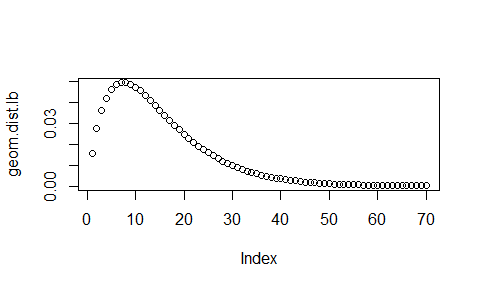# Overview of the prevtoinc package

#### 2019-06-18

require(prevtoinc)

This package implements functions to convert prevalence to incidence based on data obtained in point-prevalence studies (PPSs) along the lines of (Rhame and Sudderth 1981),(Freeman and Hutchison 1980) and is a companion to an upcoming paper (Willrich et al. 2019). It also implements methods to simulate PPS-data to benchmark different estimation methods. Notation will follow the companion paper. So a good idea is to read the paper first and afterwards the vignette.

# The basic structure of the package

The package has functions to simulate PPS-data based on distributions of length of infection and length of stay. Results of PPS simulations and incidence calculations are stored as tibbles. The functionality in the package can be divided in three parts:

• functions for PPS simulation,
• functions to estimate incidence from PPS data,
• helper functions dealing with length-biased distributions and monotone smoothing.

In the following sections, we will go through these different aspects, starting with simulation of PPS-data.

## Simulating PPS data

### Simulating a PPS

The function simulate_pps_fast can be used to generate PPS data. This functions simulates a PPS on the basis of a given prevalence P using a vector of probabilities dist.X.loi for the values 1:length(dist.X.loi) of $${{X}_{loi}}$$. It directly samples the time of infection up to date based on dist.X.loi. Optionally, the length of stay is also sampled independently using dist.X.los which is in the same format as dist.X.loi. The sample is generated by treating the marginal distributions of length of stay and length of infection as independent by assumption. Because of this non-joint sampling rows should not be interpreted as individual patients.

example.dist <- create_dist_vec(function(x) dpois(x-1, 7), max.dist = 70)
example.dist.los <- create_dist_vec(function(x) dpois(x-1, lambda = 12),
max.dist = 70)
data.pps.fast <- simulate_pps_fast(n.sample=5000,
P=0.05,
dist.X.loi = example.dist,
dist.X.los = example.dist.los)
head(data.pps.fast)
## # A tibble: 6 x 5
##   A.loi L.loi A.los L.los patient.type
##   <dbl> <dbl> <int> <int>        <dbl>
## 1     0     0     2    17            1
## 2     0     0     2     8            1
## 3     0     0     5    12            1
## 4     0     0     5    13            1
## 5     0     0    19    23            1
## 6     0     0     5    10            1

Values of zero for A.loi and L.loi indicate absence of a HAI.

The incidence rate per patient day $$I$$ and the expected length of infection in the whole population $${x_{loi}}$$ for a given distribution of $$X_{loi}$$ and given $$P$$ can be calculated with simulate_incidence_stats_fast by supplying a prevalence P and a vector of probabilities dist.X.loi for $${{X}_{loi}}$$. Optionally one can also calculate $${I_{pp}}$$ and $${{x}_{los}}$$ if one supplies a vector of probabilites dist.X.los for $${{X}_{los}}$$.

data.fast.inc.theo <- simulate_incidence_stats_fast(P=0.05,
dist.X.loi = example.dist,
dist.X.los = example.dist.los)
data.fast.inc.theo
## $x.loi ##  8 ## ##$x.los
##  13
##
## $I ##  0.006578947 ## ##$I.pp
##  0.08125

## Extended simulation method

While the above method of simulation is fast and efficient and is useful for larger simulation studies, it is useful to have a more explicit simulation technique which samples from the joint distribution of $${{X}_{los}}$$ and $${{X}_{loi}}$$ and gives more control over subpopulations of patients.

The setup of this simulation model is described in the following.

### Modelling assumptions

We assume the following setup. Patients arrive sequentially at a hospital X. A hospital is a named list with the following named elements:

• a general factor inc.factor which modifies the risk of nosocomial infections for all types of patients,
• a list of patients patient.list characterized below,
• a distribution of the patient types for the hospital given by pat.dist .

A patient.type is a list with the following named elements

• a vector dist.X.los of probabilities for the values 1:length(dist.X.los) of $${{X}_{los}}$$.,
• a vector dist.X.loi of probabilities for the values 1:length(dist.X.loi) of $${{X}_{loi}}$$.,
• and the base incidence rate (per patient-day) I.p for this type of patient.

The base-value of the length of stay is additive with the possible length of a nosocomial infection. Clustering of infections is not explicitly modelled.

### Simulation environment

As an example we define a hospital with two different patient types.

pat.1 <- list(dist.X.los =
create_dist_vec(function(x) dpois(x-1, lambda = 12),
70),
I.p = 0.008,
dist.X.loi =
create_dist_vec(function(x) dpois(x-1, lambda = 10),
70))

pat.2 <- list(dist.X.los =
create_dist_vec(function(x) dpois(x-1, lambda = 10),
70),
I.p = 0.02,
dist.X.loi =
create_dist_vec(function(x) dpois(x-1, lambda = 7),
70))

patient.list <- list(pat.1, pat.2)

# define distribution of patients
pat.1.prob <- 0.4; pat.2.prob <- 0.6
pat.dist.hosp <-  c(pat.1.prob, pat.2.prob)
hospital.1 <- list(inc.factor = 1,
pat.dist = pat.dist.hosp,
patient.list = patient.list)

Using simulate_pps_data one can generate PPS data by simulating the evolution of n.sample beds for steps days.

data.pps <- simulate_pps_data(n.sample=5000,
steps=200,
hospital=hospital.1)
head(data.pps)
## # A tibble: 6 x 5
##   A.loi A.los L.loi L.los patient.type
##   <dbl> <dbl> <dbl> <int>        <int>
## 1     0     6     0    10            1
## 2     0     7     0    10            2
## 3     0    10     0    11            1
## 4     0    11     0    14            2
## 5     9    16    11    21            1
## 6     0     4     0     8            1

To get additional theoretical quantities based on the whole population, one can use simulate_incidence_stats.

data.inc.theo <- simulate_incidence_stats(hospital.1, 365 * 1000)
# gives incidence rate I
data.inc.theo$I ##  0.01491682 # gives incidence proportion per admission data.inc.theo$I.pp
##  0.1785913
# average length of stay of patients who did not have a HAI
data.inc.theo$x.los.wo.noso ##  11.97248 # average length of stay of patients who had at least one HAI during their stay data.inc.theo$x.los.only.noso
##  17.78926

## Estimating incidence from PPS data

### The new estimator

To use the newly proposed estimator gren presented in the companion paper, one can use the function calculate_I_smooth with method="gren". The data should be supplied as a data frame with at least a column named A.loi giving lengths of infection up to date of PPS. Values of zero for A.loi indicate absence of a HAI. Optionally, the data frame can also contain a column A.los supplying lengths of stay up to PPS to estimate $${{x}_{los}}$$ with the same method as well.

calculate_I_smooth(data = data.pps,
method = "gren")
## # A tibble: 1 x 8
##       n n.noso P.hat  I.hat I.pp.hat x.loi.hat x.los.hat method
##   <int>  <int> <dbl>  <dbl>    <dbl>     <dbl>     <dbl> <chr>
## 1  5000    529 0.106 0.0125    0.149      9.45      13.3 gren
data.inc.theo$I ##  0.01491682 calculate_I_smooth(data = data.pps.fast, method = "gren") ## # A tibble: 1 x 8 ## n n.noso P.hat I.hat I.pp.hat x.loi.hat x.los.hat method ## <int> <int> <dbl> <dbl> <dbl> <dbl> <dbl> <chr> ## 1 5000 242 0.0484 0.00652 0.0771 7.81 12.4 gren data.fast.inc.theo$I
##  0.006578947

There is another variation of this estimator specified with method = "rear". This uses the rearrangement estimator studied in (Jankowski and Wellner 2009) instead of the Grenander estimator as an estimator for the monotonously decreasing distribution of $${{A}_{loi}}$$ and $${{A}_{los}}$$. We will denote this type of estimator by rear.

### Confidence intervals for the new estimator

There are two helper functions to calculate confidence intervals for the estimates of $${I_{pp}}$$ with the gren estimator: One ( calculate_CI_I_pp) is based on the typical output of calculate_I_smooth:

gren_est <- calculate_I_smooth(data = data.pps.fast, method = "gren")
gren_est
## # A tibble: 1 x 8
##       n n.noso  P.hat   I.hat I.pp.hat x.loi.hat x.los.hat method
##   <int>  <int>  <dbl>   <dbl>    <dbl>     <dbl>     <dbl> <chr>
## 1  5000    242 0.0484 0.00652   0.0771      7.81      12.4 gren
calculate_CI_I_pp(gren_est, method = "asymptotic", alpha = 0.05)
## # A tibble: 1 x 2
##   CI.lower.Ipp CI.upper.Ipp
##          <dbl>        <dbl>
## 1       0.0491        0.105

The other (CI_np_bs) is based on a bootstrapping approach which resamples from the estimates of the distributions for $${{A}_{loi}}$$ and $$Alos$$ based on the Grenander estimator. It works with data as output by simulate_pps_data*.

CI_np_bs(data.pps.fast)
## # A tibble: 1 x 2
##   CI.lower.Ipp CI.upper.Ipp
##          <dbl>        <dbl>
## 1       0.0655        0.106

### Other estimators

The function calculate_I_rhame can be used to calculate the incidence with a user-supplied value x.loi.hat for the estimated length of infection $${x_{loi}}$$ and an optional specification of x.los.hat for the estimated length of stay $${{x}_{los}}$$ to get an estimate of $${I_{pp}}$$ too. Here we take the example of an estimator where x.loi.hat and x.los.hat are fixed to their theoretical values and which depends only on the estimate of $${P}$$. We will call this type of estimator rhame.theo .

calculate_I_rhame(data.pps,
x.loi.hat = data.inc.theo$x.loi, x.los.hat = data.inc.theo$x.los,
method = "method identifier")
## # A tibble: 1 x 8
##       n n.noso P.hat  I.hat I.pp.hat x.loi.hat x.los.hat method
##   <int>  <int> <dbl>  <dbl>    <dbl>     <dbl>     <dbl> <chr>
## 1  5000    529 0.106 0.0135    0.163      8.77      13.5 method identifier
data.inc.theo$I ##  0.01491682 data.inc.theo$I.pp
##  0.1785913
calculate_I_rhame(data.pps.fast,
x.loi.hat = data.fast.inc.theo$x.loi, x.los.hat = data.fast.inc.theo$x.los,
method = "method identifier")
## # A tibble: 1 x 8
##       n n.noso  P.hat   I.hat I.pp.hat x.loi.hat x.los.hat method
##   <int>  <int>  <dbl>   <dbl>    <dbl>     <dbl>     <dbl> <chr>
## 1  5000    242 0.0484 0.00636   0.0786         8        13 method identifi~
data.fast.inc.theo$I ##  0.006578947 data.fast.inc.theo$I.pp
##  0.08125

As a convenience function, one can use calculate_I to get estimates of I for a range of estimators (including the ones studied in (Willrich et al. 2019)) based on PPS data and the accompanying theoretical data .

The estimators are the following:

• gren - new method using Grenander estimator,
• rear - new method using rearrangement estimator,
• pps.mixed - mixed estimator described in the paper,
• pps.median - estimator based on the median duration up to PPS (used in (European Centre for Disease Prevention and Control 2013) ),
• pps.mean - alternative estimator used in (European Centre for Disease Prevention and Control 2013) based on the mean instead of the median
• L.full - estimator based on samples from the PPS with information on full length of stay/infection.
• rhame.theo - estimator using theoretical values for x.loi/x.los.
  calculate_I(data.pps.fast, data.fast.inc.theo)
## # A tibble: 8 x 8
##       n n.noso  P.hat   I.hat I.pp.hat x.loi.hat x.los.hat method
##   <int>  <int>  <dbl>   <dbl>    <dbl>     <dbl>     <dbl> <chr>
## 1  5000    242 0.0484 0.00652   0.0771      7.81     12.4  gren
## 2  5000    242 0.0484 0.00778   0.0894      6.54     12.1  rear
## 3  5000    242 0.0484 0.00971   0.115       5.24     12.4  pps.mixed
## 4  5000    242 0.0484 0.0102    0.0678      5         7    pps.median
## 5  5000    242 0.0484 0.0101    0.0706      5.05      7.36 pps.mean
## 6  5000    242 0.0484 0.00619   0.0764      8.21     13.0  L.full
## 7  5000    242 0.0484 0.00636   0.0786      8        13    rhame.theo
## 8  5000    242 0.0484 0.00546   0.0667      9.31     12.8  naive

If one wants to combine the (fast) simulation step with the estimation step one can use generate_I_fast. This is just a wrapper for first calling simulate_pps_fast and then calling calculate_I.

generate_I_fast(n.sample = 10000,
P = 0.05,
dist.X.loi = example.dist,
data.theo = data.fast.inc.theo)
## # A tibble: 8 x 8
##       n n.noso  P.hat   I.hat I.pp.hat x.loi.hat x.los.hat method
##   <int>  <int>  <dbl>   <dbl>    <dbl>     <dbl>     <dbl> <chr>
## 1 10000    489 0.0489 0.00820  NA           6.27        NA gren
## 2 10000    489 0.0489 0.00820  NA           6.27        NA rear
## 3 10000    489 0.0489 0.00935  NA           5.50        NA pps.mixed
## 4 10000    489 0.0489 0.0103   NA           5           NA pps.median
## 5 10000    489 0.0489 0.0107   NA           4.81        NA pps.mean
## 6 10000    489 0.0489 0.00648  NA           7.94        NA L.full
## 7 10000    489 0.0489 0.00643   0.0795      8           13 rhame.theo
## 8 10000    489 0.0489 0.00820  NA           6.27        NA naive

## Helper functions

The function monotone_smoother implements the rearrangement estimator and Grenander estimator described in (Jankowski and Wellner 2009).

A.loi.sample <- data.pps$A.loi[data.pps$A.loi>0]
# raw histogram of data
hist(A.loi.sample)A.loi.smoothed <- monotone_smoother(A.loi.sample, method = "gren")
# estimated monotonously decreasing distribution
plot(A.loi.smoothed)For creating length-biased distributions there is length_biased_dist, which takes a vector of probabilities of a discrete positive distribution as an argument.

# geometric distribution starting in 1 and cutoff at 70 with mean at about 8.
geom.dist <- create_dist_vec(geom_dist_fct, max.dist = 70)
# calculate mean
sum(1:length(geom.dist)*geom.dist)
##  7.993895
# plot original distribution
plot(geom.dist)geom.dist.lb <- length_biased_dist(geom.dist)
# plot length biased distribution
plot(geom.dist.lb)To calculate the mean of the original distribution based on the length-biased distribution one can use length_unbiased_mean.

# length biased distribution from chunk above
length_unbiased_mean(geom.dist.lb)
##  7.993895